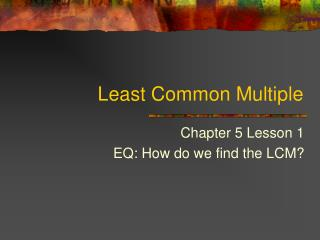DownloadDownload PresentationLeast Common Multiple

# Least Common Multiple

Download Presentation## Least Common Multiple

- - - - - - - - - - - - - - - - - - - - - - - - - - - E N D - - - - - - - - - - - - - - - - - - - - - - - - - - -
##### Presentation Transcript

1. Least Common Multiple Chapter 5 Lesson 1 EQ: How do we find the LCM?

2. Multiples • A multiple is formed by multiplying a given number by the counting numbers. • The counting numbers are 1, 2, 3, 4, 5, 6, etc.

3. Example: List the multiples of 4: • 4 x 1 = 4 • 4 x 2 = 8 • 4 x 3 = 12 • 4 x 4 = 16 • 4 x 5 = 20 • 4 x 6 = 24 So, the multiples of 4 are 4, 8, 12, 16, 20, 24, 28, etc. Counting Numbers

4. What are the first five multiples of 13? 13 x 1 =13 13 x 2 = 26 13 x 3 = 39 13 x 4 = 52 13 x 5 = 65 13, 26, 39, 52, 65

5. Find the Missing Multiples 24 30 • 6, 12, 18, ____, ____ • ___, 6, 9, 12, ____, ____, 21 • ___, 24, 36, 48, 60, ____ 3 15 18 72 12

6. Least Common Multiple (LCM) • The least common multiple is the smallest number that is common between two lists of multiples.

7. EXAMPLE: Find the LCM of 12 and 18 • The multiples of 12: • 12 x 1 = 12 • 12 x 2 =24 • 12 x 3 = 36 • 12 x 4 = 48 • 12 x 5 =60 • The multiples of 18: • 18 x 1 = 18 • 18 x 2 = 36 • 18 x 3 = 54 • 18 x 4 = 72 • 18 x 5 = 90

8. 12, 24, 36, 48, 60 18, 36, 54, 72, 90 The first number you see in both lists is 36. The least common multiple of 12 and 18 is 36.

9. Example 2: Find the LCM of 9 and 10 9, 18, 27, 36, 45, 54, 63, 72 81, 90, 99 10, 20, 30, 40, 50, 60, 70, 80 90, 100, 110 If you don’t see a common multiple, make each list go further. The LCM of 9 and 10 is 90

10. Example 3:Find the LCM of 4 and 12 4, 8, 12, 16 12, 24, 36 Answer: 12

11. Using Prime Factorization to find the LCM • Make factor trees for each number. Write the prime factorization in exponent form. • Identify all of the prime numbers among the prime factorizations. Every prime number will be used. • When the same prime number occurs in more than one prime factorization, select the prime that there’s more of (for example, 25 beats 22.) • Multiply your prime numbers to get the LCM.

12. Example: Find the LCM of 88 and 102 88 102 88 = 23 x 11 102 = 2 x 3 x 17 2 x 51 2 x 44 LCM: 23x 3 x 11 x 17 = 4,488 2 x 22 3 x 17 We have to use every prime number. The numbers we will use are 2, 3, 11, and 17. 2 x 11 We have 2 in both places. Select the “bigger” one. 23 beats regular 2.

13. Homework Time